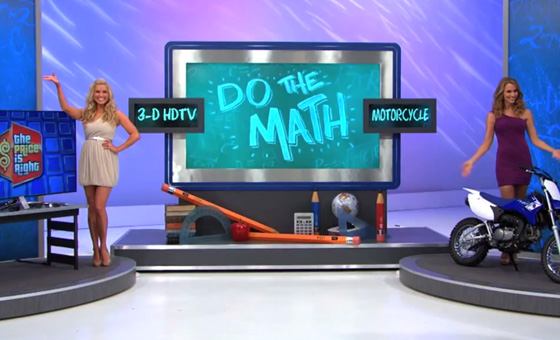Do The Math Photos & VideoVIDEO: Do The Math
Game Description In this game, the contestant has a chance to win two prizes and some money. The prizes and money are arranged in the form of a mathematical equation (using the prices of the prizes). However, the equation has both a plus sign and a minus sign in the operator position. The contestant must identify whether the plus sign or the minus sign should be used to make the equation correct. If the contestant chooses the correct operator, then the contestant wins the two prizes and the money.